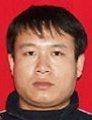Electron. J. Diff. Equ., Vol. 2013 (2013), No. 169, pp. 1-10.

### Oscillation of solutions to nonlinear forced fractional differential equations Qinghua Feng, Fanwei Meng

Abstract:
In this article, we study the oscillation of solutions to a nonlinear forced fractional differential equation. The fractional derivative is defined in the sense of the modified Riemann-Liouville derivative. Based on a transformation of variables and properties of the modified Riemann-liouville derivative, the fractional differential equation is transformed into a second-order ordinary differential equation. Then by a generalized Riccati transformation, inequalities, and an integration average technique, we establish oscillation criteria for the fractional differential equation.

Submitted April 1, 2013. Published July 26, 2013.
Math Subject Classifications: 34C10, 34K11.
Key Words: Oscillation; nonlinear fractional differential equation; forced; Riccati transformation.

An addendum was posted on November 17, 2016. It states that the Jumarie's chain rule used in equality (1.7) is incorect. See the last page of this article.

Show me the PDF file (218 KB), TEX file, and other files for this article.Qinghua Feng School of Science, Shandong University of Technology Zibo, Shandong, 255049, China email: fqhua@sina.com Fanwei Meng School of Mathematical Sciences, Qufu Normal University Qufu, 273165, China email: fwmeng163@163.com